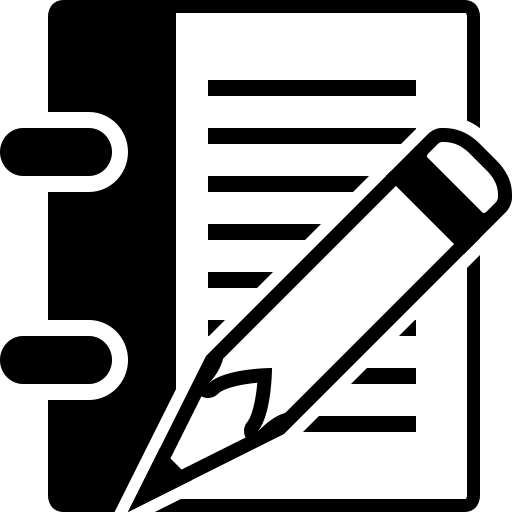## Week 13ChaosBook is a book in reverseGroup theory voodooSymmetry is your friend - overview

• 25. Deterministic diffusion / 3 April 2018 /
• Read sect. 28.2: A class of simple 1-dimensional dynamical systems where all transport coefficients can be evaluated analytically, by hand. Diffusion is a non-monotonic function of the local expansion rate, and it is non-gaussian, with a non-vanishing kurtosis. Perhaps the most fundamental diagnostic of deterministic chaos is the non-differentiable dependence of its transport coefficients on smooth variations of system parameters.Chapter 24 - Deterministic diffusionDeterministic diffusion so far - a reviewRunning orbitsCycle formulas for averagesThis is one weird formula!Diffusion on a 1-dimensional lattice

• 26. Finite groups / 5 April 2018 /
• Finite groups. Cyclic groups of two and three elements. Symmetries of a triangle, six element group multiplication table. Matrix representations. Regular representations. This is standard material, not written up in ChaosBook, but necessary for the course. We liked Tinkham, chapter 2, or Dresselhaus chapters 1 and 2 (early version available as lecture notes).Chapter xx - Finite groupsHard work builds characterThe symmetry group of a propellerIrreps of C3Rotation in the planeTrace of evolution operator??
• Homework 13
•diffusion  Due 17 April 2018Discussion forum for week 13

• Optional
•The deepest thing about chaosWhy does everybody write a book on Group Theory?Challenge: can you quotient D6 symmetry?